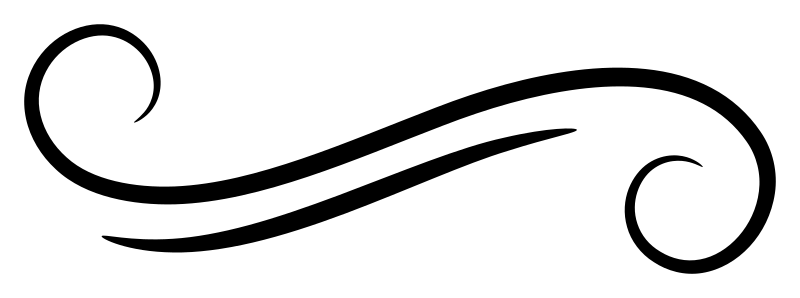# Identifiers and operators

There are two kinds of names in Haskell: Identifiers and operator symbols.

## Identifiers

An identifier starts with a letter or underscore and consists of:

• Letters
• Numbers
• `_` (underscore)
• `'` (single quote, sometimes pronounced as “prime”)

Examples of identifiers:

• `x`
• `x'``x'` is pronounced as “x prime”
• `_x`
• `π`The Greek letter pi counts as a letter.
• `player2`
• `player➆`➆ is the unicode code point U+2786, entitled “dingbat circled sans-serif digit seven,” and it is considered a number.
• `Maybe`
• `Just`
• `Functor`

It is worth noting that the underscore in isolation is a reserved identifier, used as a wildcard in patterns. In some circumstances, a lone underscore may be interpreted as a hole.

## Operator symbols

An operator symbol consists entirely of “symbol or punctuation” characters. This includes the following ASCII characters:

`!` `#` `\$` `%` `&` `*` `+` `.` `/` `<` `=` `>` `?` `@` `\` `^` `|` `-` `~` `:`

as well as a great many Unicode characters. This means we can define operators using just about any symbol we want. There’s one exception to this: There are limitations on when we can use a colon (`:`), which we discuss below. For example:

``````(<♥>) :: Integer -> Integer -> Integer
x <♥> y = x + y + 1``````
``````λ> 2 <♥> 3
6``````

## Prefix and infix

When a function is represented by an identifier, its application is typically written in prefix notation: the name of the function comes before the arguments. For example, a function named `f` applied to arguments `x` and `y` looks like this:

``f x y``

For operator symbols, function application to two arguments is typically written in infix notation: the operator comes between the arguments. For example, a function named `+` applied to arguments `x` and `y` looks like this:

``x + y``

An identifier can be used in infix notation by enclosing it in backticks. So `f x y` can be written equivalently as:

``x `f` y``

Some examples of identifiers that are conventionally used in infix notation include `mod` and `on`:

``````λ> 11 `mod` 3
2``````
``````λ> import Data.Function
λ> import Data.List

λ> sortBy (compare `on` fst) [(2, 'c'), (1, 'b'), (3, 'a')]
[(1,'b'),(2,'c'),(3,'a')]``````

An operator can be used in prefix notation by enclosing it in parentheses. So `x + y` can be written equivalently as:

``(+) x y``

## Capitalization matters

The first character of an identifier can only be a letter or an underscore, not a number or a single quote, with the underscore treated as a lowercase letter.

The following kinds of identifiers must begin with a upper case letter:

• Constructors
• Types
• Type constructors
• Type classes
• Module names

Everything else must begin with a lowercase letter or an underscore. This includes:

• Variables
• Type variables
• Functions and values other than data constructors
• Record fields

## Identifiers that end with a hash

Enabling the `MagicHash` GHC extension slightly expands the set of names that are considered valid identifiers.

If you’re going to use an operator symbol as the name of a data constructor, it must begin with a colon. Constructors are the only operators that are allowed to begin with a colon. The exception to this is type operators, which we discuss below.

For example, `NonEmpty` has a single constructor named `(:|)`.

``data NonEmpty a = a :| [a]``

Note that this rule only applies to the first character in an operator name. A colon can always appear in another position. For example:

``````(^:^) :: Integer -> Integer -> Integer
x ^:^ y = x * y + 1``````
``````λ> 3 ^:^ 4
13``````

## Operators as type names

In standard Haskell, operator symbols are only for values; you can’t use an operator as the name of a type. If you enable the `TypeOperators` GHC extension, then you can.

The rule about starting with a colon doesn’t apply at the type level. Type operators may or may not begin with a colon.

The only exception is `->`, which is a built-in type operator that you can use without the `TypeOperators` extension.Join Type Classes for courses and projects to get you started and make you an expert in FP with Haskell.